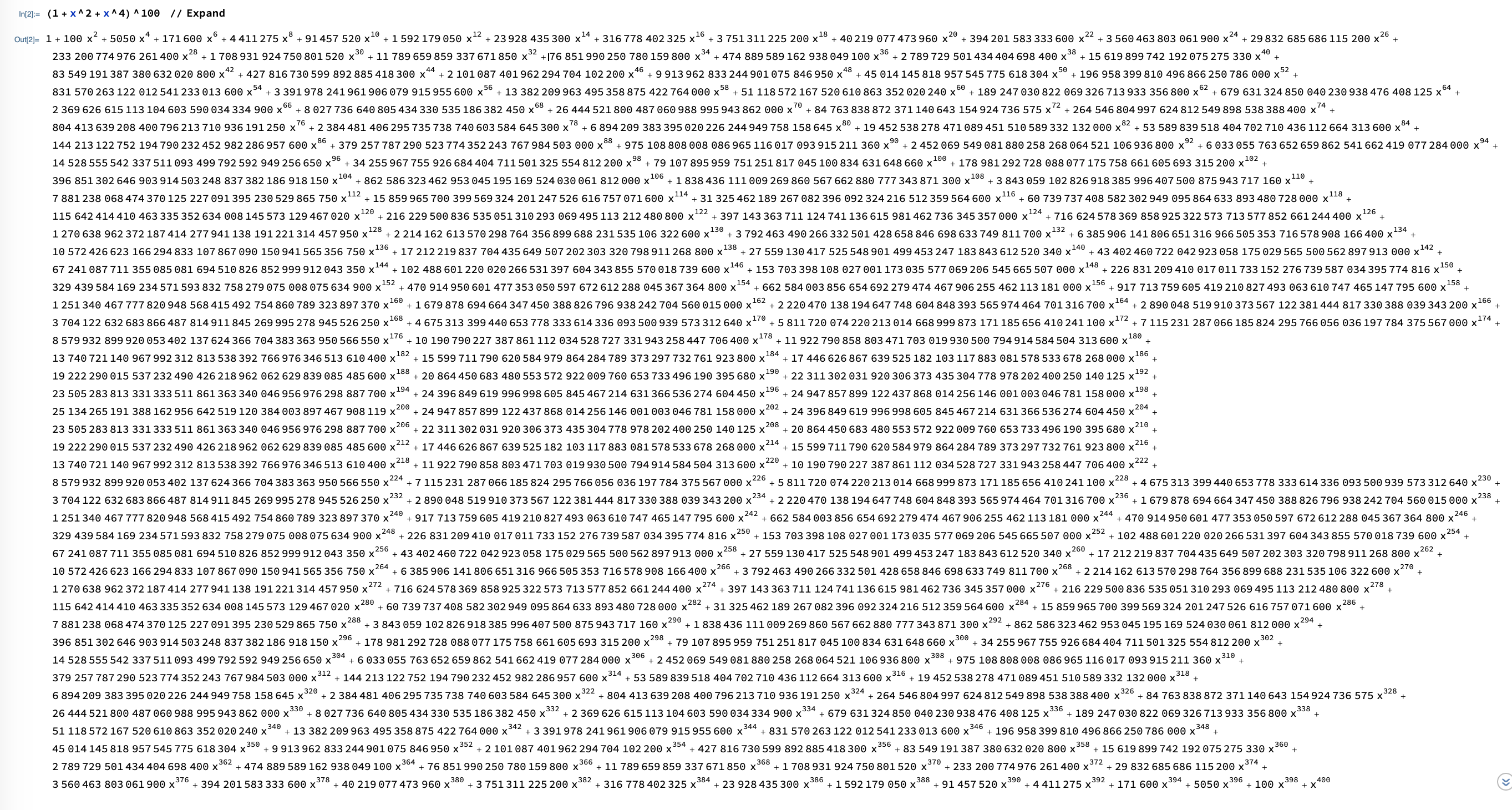# The pain rule

 The chain rule is fantastic. Avoiding it is the Pain rule. In one of the homework, you had to compute the derivative of (1+x2 + x4)100. Foiling things out gives a polynomial but is painful as the Mathematica output of ```(1+x^2+x^4)^(100) ``` illustrates. The derivative is simply 100 (1+x2+x4)99 (2x+4x3).